## New Sufficient Conditions for Hamiltonian and Traceable Graphs 111

Rao Li*

Citation: “New Sufficient Conditions for Hamiltonian and Traceable Graphs”. American Research Journal of Mathematics; V3, I1; pp:1-2.

Copyright This is an open access article distributed under the Creative Commons Attribution License, which permits unrestricted use, distribution, and reproduction in any medium, provided the original work is properly cited.

## Abstract:

In this note, we present new sufficient conditions for Hamiltonian and traceable graphs.

Keywords: Hamiltonian graph, traceable graph

## Description:

1 INTRODUCTION

We consider only finite undirected graphs without loops or multiple edges. Notation and terminology not defined here follow those in . For a graph G = (V, E), we use n and e to denote its order |V| and size |E|, respectively. The connectivity of the graph G is denoted by k(G). For disjoint subsets S, T of the vertex set V(G) of a graph G, let E(S, T) be the set of the edges in G that join a vertex in S and a vertex in T. We use G˅H to denote the join of two disjoint graphs G and H. The graph consists of p isolated vertices is denoted by Ep. A cycle C in a graph G is called a Hamiltonian cycle of G if C contains all the vertices of G. A graph G is called Hamiltonian if G has a Hamiltonian cycle. A path P in a graph G is called a Hamiltonian path of G if P contains all the vertices of G. A graph G is called traceable if G has a Hamiltonian path. In this note, we present new sufficient conditions for Hamiltonian and traceable graphs. The main results are as follows.

Theorem 1. Let G be a graph of order n ≥ 3, e edges, and connectivity k ≥ 2. If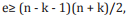then G is Hamiltonian or Kk ˅Ek+ 1, where n = 2k + 1.

Theorem 2. Let G be a graph of order n ≥ 2, e edges, and connectivity k ≥ 1. If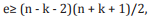then G is traceable or Kk ˅Ek+ 2, where n = 2k + 2.

Next, we will prove Theorem 1 and Theorem 2.

2 PROOFS

Proof of Theorem 1.

Let G be a graph satisfying the conditions in Theorem 1. If G has a Hamiltonian cycle, then the proof is finished. Now we assume that G is not Hamiltonian. Choose a longest cycle C in G and give an orientation on C. Since G is not Hamiltonian, there exists a vertex x0 in V(G) - V(C). By Menger’s theorem, we can find s (s ≥ k) pairwise disjoint (except for x0 ) paths P1 , P2 , ...,Ps between x0 and V(C). Let ui be the end vertex of Pi on C, where 1 ≤ i ≤ s. We, without loss of generality, assume that the appearance of u1 , u2 , ...,us on C agrees with the given orientation of C. We use ui + to denote the successor of ui along the given orientation of C, where 1 ≤ i ≤ s. Then a standard proof in Hamiltonian graph theory yields that S :={x0, u1 + , u2 + , ..., uk + } is independent (otherwise G would have cycles which are longer than C). Thus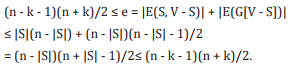Therefore |S| = k + 1, xy is in E for any vertex x in S and for any vertex y in V - S, and G[V - S)] is complete.

Let H be the component of G[V - V(C)] containing x0 . Since u1 + is adjacent to any vertex in V - S, H must consist of a singleton x0 (otherwise G would have a cycle which is longer than C). Since x0 is adjacent to any vertex in V - S, H must be the only component of G[V - V(C)] (otherwise x0 would be adjacent to a vertex in another component of G[V - V(C)], which is a contradiction). Again, since x0 is adjacent to any vertex in V - S, the segment from ui + to ui + 1 along the given orientation of C, for each i with 1 ≤ i ≤ s and s + 1 is regarded as 1, must consist of only ui + and ui + 1 (otherwise G would have a cycle which is longer than C). Hence G is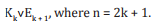This completes the proof of Theorem 1.                   QED

Proof of Theorem 2. Let G be a graph satisfying the conditions in Theorem 2. If G has a Hamiltonian path, then the proof is finished. Now we assume that G is not traceable. Choose a longest path P in G and give an orientation on P. Let y and z be the two end vertices of P. We assume that the appearance of y and z on P agrees with the given orientation of P. Since G is not traceable, there exists a vertex x0 in V(G) - V(P). By Menger’s theorem, we can find s (s ≥ k) pairwise disjoint (except for x0 ) paths P1 , P2 , ...,Ps between x0 and V(P). Let ui be the end vertex of Pi on P, where 1 ≤ i ≤ s. We, without loss of generality, assume that the appearance of u1 , u2 , ...,us on P agrees with the given orientation of P. Since P is a longest path in G, y ≠ui and z ≠ ui , for each i with 1 ≤ i ≤ s, otherwise G would have paths which are longer than P. We use ui + to denote the successor of ui along the given orientation of P, where 1 ≤ i ≤ s. Then a standard proof in Hamiltonian graph theory yields that S :={x0 , y, u1 + , u2 + , ..., uk + } is independent (otherwise G would have paths which are longer than P). Thus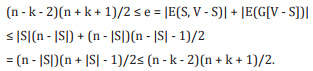Therefore |S| = k + 2, xy in E for any vertex x in S and for any vertex y in V - S, and G[V - S)] is complete.

Let H be the component of G[V - V(P)] containing x0 . Since u1 + is adjacent to any vertex in V - S, H must consist of a singleton x0 (otherwise G would have a path which is longer than P). Since x0 is adjacent to any vertex in V - S, H must be the only component of G[V - V(P)](otherwise x0 would be adjacent to a vertex in another component of G[V - V(P)], which is a contradiction). Again, since x0 is adjacent to any vertex in V - S, the segment from ui + to ui + 1 along the given orientation of P, for each i with 1 ≤ i ≤ s–1, must consist of only ui + and ui + 1 (otherwise G would have a path which is longer than P). Moreover, the segment from y to u1 along the given orientation of P must consist of only y and u1 and the segment from us + to z along the given orientation of P must consist of only us + . Hence G is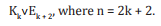This completes the proof of Theorem 2.                  QED

## References

1. J. A. Bondy and U. S. R. Murty, Graph Theory with Applications, Macmillan, London and Elsevier, New York (1976).

## Testimonials### Chicago

• 8770 West Bryn Mawr Ave, Suite 1300
Chicago, Illinois - 60631-3515, USA.
• +1-773-649-9077
• +1-479-402-4700

### Arkansas

• 1120 S Walton Blvd, Suite 138
Bentonville, Arkansas - 72712-0077, USA.
• +1-479-696-9120
• +1-479-696-9132